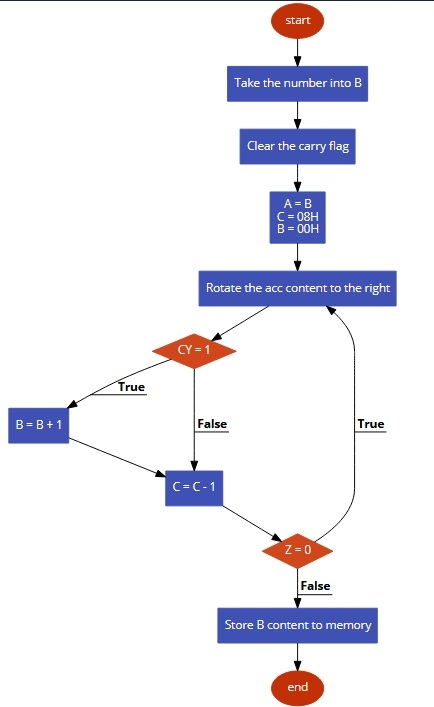# 8085 program to count the number of ones in contents of register B

In this program, we will see how to count the number of 1’s in an 8-bit number stored in register B.

## Problem Statement

Write 8085 Assembly language program to count the number of 1s in 8-bit number stored in register B.

## Discussion

In this program, we are using the rotate operation to count the number of 1’s. As there are 8 different bits in 8-bit number, then we are rotating the number eight times. we can use RRC or RLC. Here we have used the RRC instruction. This instruction sends the LSb to MSb also to carry flag. So after each iteration, we can check the carry status to get the count of 1s.

If the number is DA (1101 1010) then the answer will be 5, as there are five 1s in the number.

Register
Data
B
DA

## Flow Diagram## Program

HEX Codes
Labels
Mnemonics
F000
06, DA

MVI B, DA
Take number 1101 1010
F002
37

STC
Set Carry
F003
3F

CMC
Complement Carry
F004
78

MOV A,B
Load the number into A from B
F005
0E, 08

MVI C,08H
Initialize the counter to 08H
F007
06, 00

MVI B,00H
Clear B register
F009
0F
LOOP
RRC
Rotate right
F00A
D2, 0E, F0

JNC SKIP
If CY = 0, skip the next step
F00D
04

INR B
If CY = 1, increase B
F00E
0D
SKIP
DCR C
Decrease C by 1
F01F
C2, 09, F0

JNZ LOOP
F012
78

MOV A,B
F013
32, 50, 80

STA 8050H
Store the result at 8050H
F016
76

HLT
Terminate the program